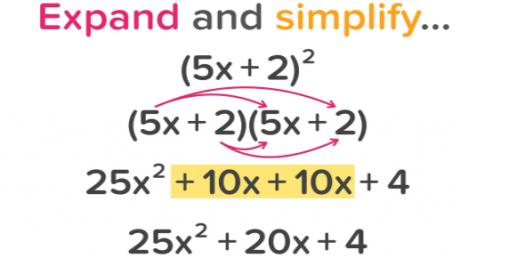4 Questions | Total Attempts: 28SettingsCombine like terms and use the order of operations to simplify algebraic expressions.

Related Topics
• 1.
3x + 1 + 8x + 9
• A.

12x + 10

• B.

5x + 8

• C.

11x + 11

• D.

11x + 10

• 2.
2x + 5y - 7x + 8y
• A.

9x + 13y

• B.

5x + 3y

• C.

-5x + 3y

• D.

-5x + 13y

• 3.
10x + 14x + 15y + 10y
• A.

24x + 15y

• B.

10 x + 25 y

• C.

24x + 25y

• D.

None of the above